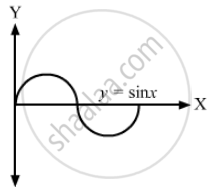Department of Pre-University Education, KarnatakaPUC Karnataka Science Class 11

# A Curve is Represented by Y = Sin X. If X is Changed from π 3 T O π 3 + π 100 , Find Approximately the Change in Y. - Physics

Sum

A curve is represented by y = sin x. If x is changed from $\frac{\pi}{3}\text{ to }\frac{\pi}{3} + \frac{\pi}{100}$ , find approximately the change in y.

#### Solution

y = sin x   ...(i)Now, consider a small increment ∆x in x.
Then y + ∆y = sin (x + ∆x)   ...(ii)
Here, ∆y is the small change in y.

Subtracting (ii) from (i), we get:
∆y = sin (x + ∆x) − sin x
$= \sin \left( \frac{\pi}{3} + \frac{\pi}{100} \right) - \sin \frac{\pi}{3}$
= 0.0157

Concept: What is Physics?
Is there an error in this question or solution?
Chapter 2: Physics and Mathematics - Exercise [Page 29]

#### APPEARS IN

HC Verma Class 11, Class 12 Concepts of Physics Vol. 1
Chapter 2 Physics and Mathematics
Exercise | Q 21 | Page 29
Share